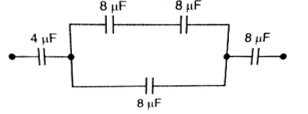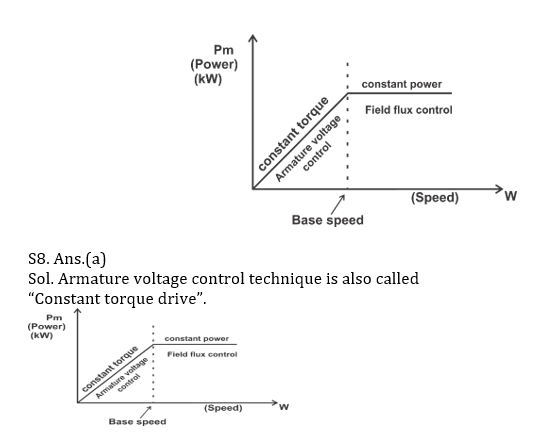Engineering Jobs   »   DFCCIL ELECTRICAL QUIZ

# DFCCIL’21 EE: Weekly Practices Quiz 08-Aug-2021

Each question carries 1 mark.
Negative marking: 1/4 mark
Total Questions: 12
Time: 12 min.

Q1. The type of single-phase induction motor having the highest power factor at full load is
(a) Capacitor run type
(b) Capacitor start type
(d) Split Phase Type

Q2. A single winding single-phase induction motor has
(a) Low starting torque
(b) Zero starting torque
(c) High starting torque
(d) Starting torque equal to full-load torque

Q3. The phasor current through an impedance is 1∠0° A and the voltage across it is 2∠60° V. The reactive power in VARs consumed in the impedance is
(a) j√(3/2)
(b) -j√(3/2)
(c) j√3
(d) – j√3

Q4. A 20 kVA, 2000 V/200 V, 2-winding transformer, when used as an autotransformer with constant voltage source of 200 V, is capable of handling
(a) 20 kVA/ 20 kVA
(b) 220 kVA/ 220 kVA
(c) 320 kVA/ 320 kVA
(d) None of the above

Q5. Two resistors of resistances 8 Ω and 12 Ω are connected in parallel. The power dissipated in 8 Ω resistor is 9 W. The power dissipated is 12 Ω resistor is
(a) 12 W
(b) 24 W
(c) 6 W
(d) 16 W

Q6. The equivalent capacitance between points A and B in the circuit shown in Fig. below is(a) 3 μ F
(b) 6.2 μ F
(c) 4.18 μ F
(d) 2.18 μ F

Q7. Field control of a DC shunt motor gives–
(a) Constant kW drive
(b) Constant torque drive
(c) Constant speed drive

Q8. In a DC motor, speed control by varying the armature circuit resistance provides a:
(a) constant torque drive
(b) variable torque drive
(c) constant power drive
(d) variable power drive

Q9. The r.m.s. value of an a.c wave is always greater than the average value except in
The case of ……. wave.
(a) sawtooth and triangular
(b) semi-circular
(c) square and rectangular
(d) none of the above

Q10. With feedback……………reduces?
(a) System stability
(b) System gain
(c) Both a and b
(d) None of the above

Q11. The topmost wire in distribution systems is:
(a) Neutral wire
(b) Phase wire
(c) Earth wire
(d) None of these

Q12. In a transformer the voltage regulation will be zero when it operates at:
(a) Lagging pf
(c) Unity pf

SOLUTIONS

S1. Ans.(a)
Sol. In capacitor run motor the starting winding remains connected all the times. Because the capacitor remains in the circuit all the time a lower value capacitor is used the lower value capacitor has more phase shift as compared to the high-value capacitor, therefore, winding current is low and it has high running torque and improved power factor.

S2. Ans.(b)
Sol. A single phase induction motor consists of a single-phase winding on the stator and a cage winding on the rotor. When a single-phase supply is connected to the stator winding, a pulsating magnetic field is produced. Therefore, a single-phase induction motor is not self-starting and requires some particular starting means.

S3. Ans.(d)
Sol. Complex power is S= P+JQ, where Q is negative for lagging power factor and positive for leading power factor.
Q=V_rms I_rms sinϕ
= 2×1×sin 60⁰
= j√3
But here current is lagging behind voltage by 60⁰. So, Q will be negative. i.e. -j√3.

S4. Ans.(b)
Sol. (in case of transformer,we know∶→)/((VI)input=(VI)output)
For auto-transformer a = 2000/200=10
∵Sauto=(a+1) S_2wdg
(10+1)×20=220 KVA

S5. Ans.(c)
Sol. P=V^2/R or V=√(PR.) Since voltage is same in a parallel circuit, P_1 R_1=P_2 R_2 or 9×8 = P_2×12
∴P_2=6 W

S6. Ans.(d)
Sol. C_eq=(4×12×8)/(4×12+12×8+4×8) = 2.18 μ F

S7. Ans.(a)
Sol. There are 3 types of speed control for dc drives: –
(I.) Armature voltage control
(ii) Field flux control
(iii) Armature resistance control
We know▭(N∝1/ϕ)
Armature voltage control technique gives high efficiency but below base speed.
So, above base speed, Field flux control is used, where by weakening the field, speed above base speed can be achieved because these two are inversely proportional to each other.S9. Ans.(c)
Sol. In case of rectangular and symmetrical square wave, r.m.s. value is equal to the average value. In case of all other a.c waves, r.m.s. value is greater than the average value.

S10. Ans.(b)
Sol. With positive feedback, system gain reduces.

S11. Ans.(c)
Sol.
The topmost wire in distribution systems is Earth wire.
Earth wire is used for protection against lightning.

S12. Ans.(b)
Sol. In a transformer the voltage regulation will be zero when it operates at leading pf.

▭(VR=(%R)cosϕ\ ±(%X)sinϕ)
+ → for lagging